# Field Emission

(redirected from Fowler-Nordheim tunneling)
Also found in: Dictionary, Thesaurus, Acronyms.

## Field emission

The emission of electrons from a metal or semiconductor into vacuum (or a dielectric) under the influence of a strong electric field. In field emission, electrons tunnel through a potential barrier, rather than escaping over it as in thermionic or photoemission. The effect is purely quantum-mechanical, with no classical analog. It occurs because the wave function of an electron does not vanish at the classical turning point, but decays exponentially into the barrier (where the electron's total energy is less than the potential energy). Thus there is a finite probability that the electron will be found on the outside of the barrier. See Photoemission, Quantum mechanics, Thermionic emission

For a metal at low temperature, the process can be understood in terms of the illustration. The metal can be considered a potential box, filled with electrons to the Fermi level, which lies below the vacuum level by several electronvolts. The distance from Fermi to vacuum level is called the work function, &phgr;. The vacuum level represents the potential energy of an electron at rest outside the metal, in the absence of an external field. In the presence of a strong field, the potential outside the metal will be deformed along the line AB, so that a triangular barrier is formed, through which electrons can tunnel. Most of the emission will occur from the vicinity of the Fermi level where the barrier is thinnest. See Electron emission

McGraw-Hill Concise Encyclopedia of Physics. © 2002 by The McGraw-Hill Companies, Inc.
The following article is from The Great Soviet Encyclopedia (1979). It might be outdated or ideologically biased.

## Field Emission

(or cold emission), the emission of electrons by a solid or liquid conductor under the action of an external electric field E of high strength. For field emission to occur, E must be at least ~107 volts per centimeter (V/cm).

Field emission was first observed in 1897 by R. Wood of the USA. In 1929, R. Millikan and C. Lauritsen established that the logarithm of the field emission current density j is linearly dependent on the reciprocal 1/E of the electric field strength. In 1928 and 1929, R. Fowler and L. Nordheim put forth a theoretical explanation of field emission on the basis of the tunnel effect. Field emission is a result of the tunneling of electrons through the potential barrier that exists at the interface between a conductor and a vacuum or other medium. A strong electric field lowers the barrier and makes it sufficiently penetrable, that is, relatively thin and low. Field emission is sometimes called autoelectronic emission because in it, in contrast to other types of electron emission, energy is not expended to excite the electrons. In the Soviet literature, field emission is often referred to as tunnel emission (tunnel’naia emissiia).

The field emission current density j is part of the flux density n of electrons incident on the barrier from inside the conductor and is determined by the transmission coefficient D of the barrier: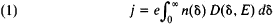Here, 8 is the fraction of the electron’s energy that is associated with the component of momentum normal to the surface of the conductor, E is the electric field strength at the surface, and e is the electron charge. It follows from equation (1) that y is dependent on the electron concentration in the conductor and the electron energy spectrum and on the height and shape of the barrier, which determine D.

Field emission from metals into a vacuum has been studied most thoroughly. In this case the Fowler-Nordheim formula holds: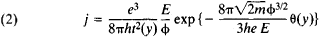Here, h is Planck’s constant, m is the mass of the electron, φ is the work function of the metal, and t(y) and θ(y) are functions of the variable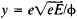(values of the functions can be obtained from tables). By inserting the values of the constants and assuming t2(y) ≈ 1.1 and θ(y) = 0.95–1.03 y2, we obtain from equation (2) the approximate relation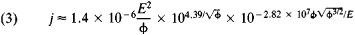where the quantities j, E, and φ are given in amperes per cm2(A/cm2), V/cm, and electron volts (eV) respectively. The values of log; for some E and φ are given in Table 1.

Equation (2) was obtained on the assumptions that the temperature is T = 0°K and that only image forces act on the electrons outside the metal in the absence of a field (seeWORK FUNCTION). The shape of the potential barrier for this case is shown in Figure 1. The transmission coefficient D of the barrier can be calculated

Table 1. Values of log j for certain values of Eand φ
φ = 20φ = 45φ = 8.3
E × 10–7log jE × 10–7log jE × 10–7log j
1.02.982.0–3.332.0–12.90
1.24.453.01.574.0–0.88
1.45.494.04.066.03.25
1.66.275.05.598.05.34
1.86.896.06.6210.06.66
2.07.407.07.3612.07.52
2.27.828.07.9414.08.16
2.48.169.08.3916.08.65
2.68.4510.08.7618.09.04
12.09.3220.09.36

by means of the Wentzel-Kramers-Brillouin method. Despite its simplifications, the Fowler-Nordheim theory is in good agreement with experiment.

In practice, measurements are usually made of the dependence of the current I = jS on the voltage V: E = αV. Here, S is the area of the emitting surface, and α is the field form factor. Field emission from metals is characterized by maximum current densities of up to j ~ 1010 A/cm2. This circumstance is explained by the Fowler-Nordheim theory. Deviations from equation (2) occur only at j ~ 106-109 A/cm2; they are due to the influence of the space charge or the shape of the potential barrier near the surface of the metal. When/ ~ 108—1010 A/cm2, an unrestricted increase in voltage leads to electrical breakdown of the vacuum gap, after an intense, brief explosive emission of electrons, and to the destruction of the emitter.

Field emission is weakly temperature dependent. Small deviations from equation (2) with increasing temperature T are proportional to T2: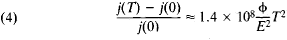Equation (4) is correct to within 1 percent for current increments <18 percent. For larger changes in current, more cumbersome formulas and computer-calculated curves are used. With rising temperature and decreasing E, temperature-and-field, or T-F, emission gives way to field-enhanced thermionic emission (the Schottky effect).

The energy spectrum of the electrons leaving the metal in field emission is narrow (Figure 2, page 56.). The half-width σ of the distribution of electrons with respect to total energies & (in eV) at T = 0°K is given by the equation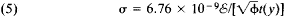When φ = 4.4 eV, σ varies from 0.08 to 0.2 eV for changes in j from 0 to 7. With increasing T, σT increases; in particular, at 300°K (given the same variation of j) σT varies from 0.17 to 0.3 eV. The character of the energy distribution of the electrons deviates from the theoretical distribution when the Fermi surface has a complex configuration or when adsorbed atoms (especially nonmetallic atoms) are present on the surface of the metal. If adsorbed organic molecules or complexes thereof are present on the surface of the metal, the electrons pass through them, and they act as wave guides for the corresponding de Broglie waves. The observed distributions of electron density over the waveguide cross section here are distributions typical of wave guides. The energy spectra of the electrons in this case exhibit anomalies.

The drawing of current at low temperatures causes heating of the emitter, since the outgoing electrons carry off energy that is, on the average, less than the Fermi level, whereas the energy of electrons entering the metal is precisely at this level. As T increases, the opposite effect is observed: heating is replaced by cooling when some temperature corresponding to a symmetric (with respect to the Fermi level) distribution of the outgoing electrons over the total energies is passed. At high currents, when the emitter is heated by Joule heat, this cooling effect to some extent impedes avalanche self-heating and stabilizes the field emission current.

Field emitters are made in the form of surfaces of large curvature, such as needle points or shape edges. In the case, for example, of needle points with a radius of curvature of 0.1–1 micrometer, a voltage of ~1–10 kilovolts is usually sufficient to create a field E ~ 107 V/cm at the surface of the point. Multiple-point emitters are used to produce high currents.

The stability of a field emission current is ensured by the constancy of the distribution of φ and α along the surface of the emitter. Both quantities may vary under the influence of the adsorption and migration of atoms of foreign substances and the material of the emitter. Local values of α increase as the material of the surface migrates in the presence of a strong electric field. In the cathode-anode space and at the surface of the anode, the electron beam creates positive ions that bombard the emitter and destroy its surface. Consequently, the stability of field emission can be increased in the following ways: by improving the vacuum and cleaning the electrodes, by using pulsed voltage, and by moderately heating the emitter to protect it from adsorption of residual gases and to smooth out defects at the points of ion impact. Field emission from single crystals of practically all refractory metals and of such chemical compounds with metallic electrical conduction as ZrC and LaB6 has been investigated in an ultra-high vacuum, where the surface of the emitter remains pure for hours or days. Field emission from W, Mo, and Re has been studied most thoroughly.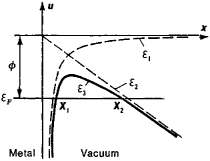Figure 1. Potential energy u of an electron near the surface of a metal: (x) distance of electron from surface, (ε1) potential energy in the absence of an electric field, (ε2) potential energy in a uniform external electric field, (ε3) total potential energy of electron, (εF) Fermi level of metal, (X2X1) width of potential barrier in the presence of an electric field

Applications of field emission from metals are associated with the possibility of obtaining high currents or intense electron beams. Cold metal cathodes show considerable promise in high-current devices and are in fact used for obtaining X-ray flashes or electron beams that can be sent outside the tube through a thin foil, for pumping in masers, and for shaping electron bunches in the collective acceleration of heavy ions. The nonlinearity of the volt-ampere characteristic of field-emission devices is made use of in frequency amplifiers and mixers, microwave signal amplifiers and detectors, and other devices. The field emitter is used as an intense point source of electrons in scanning electron microscopes. It has promising applications in X-ray and electron microscopy, in X-ray microanalyzers, and high-resolution electron-beam instruments. Field-emission cathodes also show promise in microelectronics as sensitive detectors of voltage changes. Field emission from a metal into a dielectric is also of great importance (seeDIELECTRIC ELECTRONICS). The field-emission electron microscope consists of a field emitter and an anode combined with a fluorescent screen. The angular distribution of field-emission electrons from a point can be observed on the screen at a magnification of ~105-106 and a resolution of 20–60 angstroms.

Field emission from semiconductors has been studied less thoroughly. It is characterized by more complicated dependences of j on E and φ and on the energy spectra of the electrons. In field emission from semiconductors the electric field, on penetrating into the crystal, displaces the energy bands and locally alters charge carrier concentrations and energy distributions. Further-more, the conduction electron concentration is lower in semiconductors than in metals. This circumstance limits the value of j. External factors that strongly influence the electron concentration, such as temperature and illumination, also appreciably alter j. The current-voltage relations j (E) and the energy spectra of the electrons reflect the band structure of semiconductors. A current flowing through a semiconductor may redistribute the potential in a specimen and influence the electron energy distribution.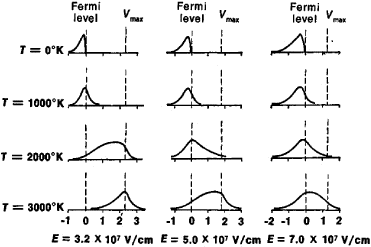Figure 2. Energy spectra of electrons emitted in field emission for different temperatures and electric fields when φ = 4.5 eV: (T) temperature, (E) electric field strength

Semiconductor field emitters that react to light are promising as sensitive detectors of infrared radiation. Multiple-point systems of such emitters can serve as the basis for mosaic systems in infrared image converters. In some cases, when the current-voltage characteristics of a semiconductor are determined entirely by its volumetric properties, j is weakly dependent on E and φ. In such cases an unheated point source of electrons can operate stably for a long period in a relatively low vacuum.

### REFERENCES

Wood, R. W. Phys. Rev., 1897, vol. 5, no. 1.
Millikan, R. A., and C. C. Lauritsen. Phys. Rev., 1929, vol. 33, no. 4, p. 598.
Fowler, R. H., and L. Nordheim. Proc. Roy. Soc., series A, 1928, vol. 119, no. 781, p. 173.
Nordheim, L. Phys. Zs., 1929, no. 7, p. 177.
Elinson, M. I., and G. F. Vasil’ev. Avtoelektronnaia emissiia. Moscow, 1958.
Nenakalivaemye katody. Edited by M. I. Elinson. Moscow, 1974.
Fischer, R., and H. Neumann. Avtoelektronnaia emissiia polupro-vodnikov. Moscow, 1971. (Translated from German.)

V. N. SHREDNIK

## field emission

[′fēld ə‚mish·ən]
(electronics)
The emission of electrons from the surface of a metallic conductor into a vacuum (or into an insulator) under influence of a strong electric field; electrons penetrate through the surface potential barrier by virtue of the quantum-mechanical tunnel effect. Also known as cold emission.
McGraw-Hill Dictionary of Scientific & Technical Terms, 6E, Copyright © 2003 by The McGraw-Hill Companies, Inc.
References in periodicals archive ?
Because Fowler-Nordheim tunneling involves only the programming current flow, not a relatively large source-to-drain current as well, it is very power efficient, NAND flash uses tunneling for both programming and erasing and has the advantage of fast programming because low-power programming allows many cells to be programmed simultaneously.
Depending on the oxide voltage level, injection current in Si[O.sub.2] is due to direct tunneling through a trapezoidal barrier or due to Fowler-Nordheim tunneling trough a triangular barrier.
At the same gate bias where Fowler-Nordheim tunneling is dominating, the electron tunneling distance from Si-substrate to the conduction band of the storage dielectric is therefore shorter for structures with [Ta.sub.2][O.sub.5]/Si[O.sub.2].

Site: Follow: Share:
Open / Close